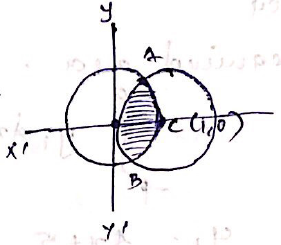# Find the area of the region bounded by the curvesusing integration.Thus,
center=(1,0)Thus
center=(0,0)
Area required = Area  OACB
First, we find Intersection
points A & BFrom equation (1)putin equation (2)putingin equation (1)